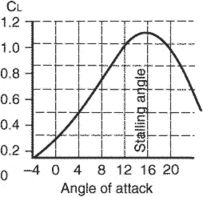# lift coefficient

Also found in: Wikipedia.

## lift coefficient

[′lift ‚kō·i‚fish·ənt]
(aerospace engineering)
The quantity CL = 2 LV 2 S, where L is the lift of a whole airplane wing, ρ is the mass density of the air, V is the free-stream velocity, and S is the wing area; this is also applicable to other airfoils.
McGraw-Hill Dictionary of Scientific & Technical Terms, 6E, Copyright © 2003 by The McGraw-Hill Companies, Inc.

## lift coefficientRelationship between angle of attack and lift coefficient.
A dimensionless number used in the formulae for aerodynamic lift that varies with the angle of attack (α) and the shape of the airfoil. The coefficient is derived from wind tunnel data and describes the characteristics of an airfoil.
References in periodicals archive ?
9, b, as the height from the ground decreased, the lift coefficient diminished to some small value due to the influences of the suction area developed on the lower surface of the wings.
The powered-lift, developed by researchers at the NASA Langley Research Center in the mid-1950s, gave the aircraft close to double the lift coefficient of a conventional transport airframe by positioning the engines and flaps in a way that directed the exhaust downward.
Based on the averaged drag and lift coefficient an AL model for the tower was implemented.
Thirdly, the maximum mean lift coefficient as well as hovering efficiency is achieved when the wing has [[rho].sup.*] = 1750, which indicates that the flexible wing with [[rho].sup.*] = 1750 has better hovering performance than the other considered wing in this section.
Although the maximal lift coefficient is low comparing to other conceptual studies , especially dedicated for the same purpose , the designed UAV has satisfactory parameters from the project aims point of view.
The Lift Coefficient (Cl) is the lifting force and Drag Coefficient (Cd) is the drag or resistance force that generated by the airfoils are important role in designing wind turbine.
Table 1: Characteristic design Parameters Chord 0.4167 ft Lift Curve Slope 4.712 /rad Min Lift Coefficient -1.1 Max Lift Coefficient 1.1 Drag Coefficient Gain 0.9 Drag Coefficient Offset 0.9 Duct Moment Coefficient 0.8 Table 2: Mesh details Mesh type Hybrid mesh (tetra/prism) Global element size 0.04 Prism mesh 0.004 Prism mesh layers around duct 6 Total mesh elements 5,48,446 Mesh nodes 95,317 Table 3: Result Analysis L/D ratio for duct 3.686 L/uD ratio for ducted propeller 3.862
The aerodynamic characteristic parameters of the ice-coated conductor include drag coefficient, lift coefficient, and torsional coefficient.
When the Reynolds numbers are 1000 and 30000, respectively, changing L/D = 0.5~2.0 to simulation can be found to reduce the drag coefficient, lift coefficient, and vortex shedding frequency.
According to the time-averaged value of the calculated data, the relations between drag coefficient, lift coefficient, and angle of attack are depicted in Figure 5.

Site: Follow: Share:
Open / Close